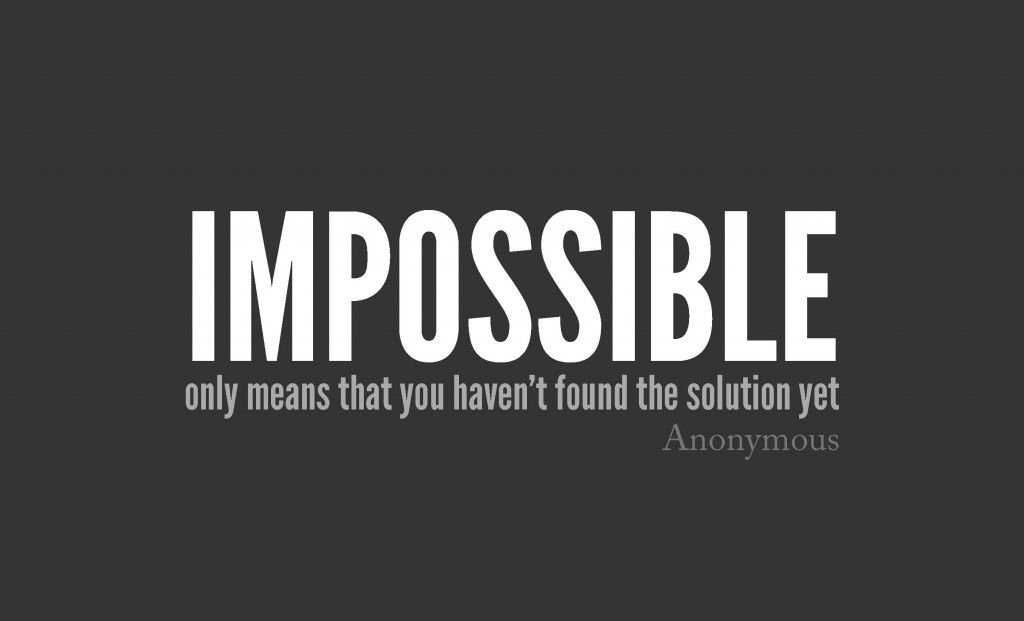## Do something impossible before breakfast

Given: a = b Multiply by a: a2 = ab Subtract b2: a2 – b2 = ab – b2 Factorise: (a + b)(a – b) = b(a – b) Divide by (a – b): (a + b) = b Substitute for b: a + a = a Simplify: 2a = a Divide by a: 2 = 1 Clearly, this is impossible. So...